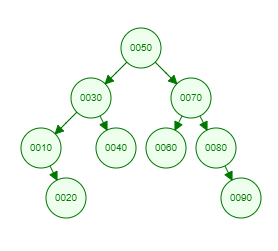Recent Tutorials and Articles
Implementing Pre-Order Traversal of Binary Tree in Java
Published on: 27th October 2018

This tutorial provides code for implementing pre-order traversal of a Binary Tree.

# Pre-Order Traversal of Binary Tree

Binary Tree traversal is a process of visiting each node of Binary Tree exactly once. Pre-Order traversal falls into depth-first search as search is deepended on child nodes of a node before moving to its sibling.

Pre-order traversal is a special type of Tree traversal wherein node data is printed before its left child node data followed by right child node data. This results into recursive function calls as you can't print a right child node data unless all of its left node data have been printed.

Let's consider below Binary Tree -Here is the Pre-Order Traversal of above tree -

``````50 30 10 20 40 70 60 80 90
``````

# Java Implementation of Pre-Order Traversal of Binary Tree

Here is Java code for Binary Tree containing methods for inserting data and pre-order traversal -

``````package com.aksain.data.structures;

public class BinaryTreeWithPreOrderTraversal<T extends Comparable<T>> {
private Node root;

private class Node {
T data;
Node left;
Node right;

private Node(T data) {
this.data = data;
}
}

private Node doInsert(T data, Node node) {
if(node == null) {
return new Node(data);
}
int result = data.compareTo(node.data);
if(result < 0) {
node.left = doInsert(data, node.left);
} else if(result > 0) {
node.right = doInsert(data, node.right);
}

return node;
}

public void insert(T data) {
root = doInsert(data, root);
}

private void doPreOrder(Node node) {
if(node == null) {
return;
}
System.out.print(node.data + " ");
doPreOrder(node.left);
doPreOrder(node.right);
}

public void preOrder() {
doPreOrder(root);
System.out.println();
}

public static void main(String[] args) {
final BinaryTreeWithPreOrderTraversal<Integer> binaryTree = new BinaryTreeWithPreOrderTraversal<>();
binaryTree.insert(50);
binaryTree.insert(30);
binaryTree.insert(10);
binaryTree.insert(20);
binaryTree.insert(40);
binaryTree.insert(70);
binaryTree.insert(60);
binaryTree.insert(80);
binaryTree.insert(90);

binaryTree.preOrder();
}
}
``````

Here is the output of above code -

``````50 30 10 20 40 70 60 80 90
``````

Thank you for reading through the tutorial. In case of any feedback/questions/concerns, you can communicate same to us through your comments and we shall get back to you as soon as possible.

Published on: 27th October 2018